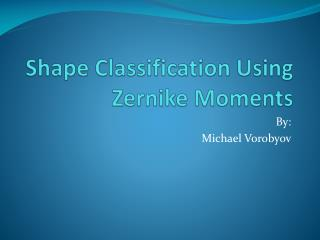DownloadDownload PresentationShape Classification Using Zernike Moments

# Shape Classification Using Zernike Moments

Download Presentation## Shape Classification Using Zernike Moments

- - - - - - - - - - - - - - - - - - - - - - - - - - - E N D - - - - - - - - - - - - - - - - - - - - - - - - - - -
##### Presentation Transcript

1. Shape Classification Using Zernike Moments By: Michael Vorobyov

2. Moments • In general, moments are quantitative values that describe a distribution by raising the components to different powers

3. Regular (Cartesian) Moments • A regular moment has the form of projection of onto the monomial

4. Problems of Regular Moments • The basis set is not orthogonal  The moments contain redundant information. • As increases rapidly as order increases, high computational precision is needed. • Image reconstruction is very difficult.

5. Benefits of Regular Moments • Simple translational and scale invariant properties • By preprocessing an image using the regular moments we can get an image to be translational and scale invariant before running Zernike moments

6. Orthogonal Functions • A set of polynomials orthogonal with respect to integration are also orthogonal with respect to summation.

7. Orthogonal Moments • Moments produced using orthogonal basis sets. • Require lower computational precision to represent images to the same accuracy as regular moments.

8. Zernike Polynomials • Set of orthogonal polynomials defined on the unit disk.

9. Zernike Moments • Simply the projection of the image function onto these orthogonal basis functions.

10. Advantages of Zernike Moments • Simple rotation invariance • Higher accuracy for detailed shapes • Orthogonal • Less information redundancy • Much better at image reconstruction (vs. cartesian moments) VS.

11. Scale and Translational Invariance Scale: Multiply by the scale factor raised to a certain power Translational: Shift image’s origin to centroid (computed from normal first order moments)

12. Rotational Invariance The magnitude of each Zernike moment is invariant under rotation.

13. Image Reconstruction • Orthogonality enables us to determine the individual contribution of each order moment. • Simple addition of these individual contributions reconstructs the image.

14. Can you guess the reconstruction?

15. Order: 5 • Ball? • Face? • Pizza?

16. Order: 15 • Squirrel? • Mitten? • Cowboy Hat?

17. Order: 25 • Dinosaur? • Bird? • Flower?

18. Order: 35 • Bird? • Plane? • Superman?

19. Order: 45 • Pterodactyl? • Crane? • Goose?

20. Crane!

21. Image Reconstruction Reconstruction of a crane shape via Zernike moments up to order 10k-5, k = {1,2,3,4,5}. (a) (b) (c) (d) (e) (f)

22. Determining Min. Order After reconstructing image up to moment • Calculate the Hamming distance, which is the number of pixels that are different between and • Since, in general, decreases as increases, finding the first for which will determine the minimum order to reach a predetermined accuracy.

23. Experimentation & Results • We used a leaf database of 62 images of 19 different leaf types which were reduced to a 128 by 128 pixel image from 2592 by 1728 pixel image • Made to be scale and translational by resizing to a common area of 1450 pixels and putting the origin at the centroid • Clustering was done by using the Hierarchical clustering method

24. Image Database

25. Original Clusters Type 1 Type 5 Type 6 Type 2 Type 7 Type 3 Type 4

26. The Zernike Clusters Type 1 Type 5 Type 2 Type 6 Type 7 Type 3 Type 4

27. How to Evaluate Clusters • Cue validity is a measure of how valid is the clustering with respect to the cue or object type. • Category validity is the measure of how valid is the clustering with respect to other inter-cluster objects. • Combination

28. Cue/Category Validity Cue: max(4/6, 1/7, 1/6) = 4/6 Cat: max(4/6, 1/6, 1/6) = 4/6 Q = 4/6 * 4/6 = 4/9 Cue: max(2/6, 1/7, 5/6) = 5/6 Cat: max(2/8, 1/8, 5/8) = 5/8 Q = 5/6 * 5/8 = 25/48 Cue: max(0/6, 5/7, 0/6) = 5/7 Cat: max(0/5, 5/5, 0/5) = 5/5 Q = 5/7 * 5/5 = 5/7

29. Clustering Results for Different Orders

30. How To Improve Results? • High order Zernike Moments are computed to really high powers they capture a lot of noise • Propose 4 weight functions • Polynomial: • Exponential: • Order Amount: where • Hamming Distance: where

31. Weight Functions

32. Results

33. Results Cont.

34. Conclusion • The ideal number of moments to use in a clustering problem depends on the data at hand and for shapes, nearly all the information is found in the boundary. • For our dataset, we found that by utilizing a high number of moments becomes redundant • For our data ZM's seem to reach an optimal accuracy at around the order 15 and afterwards seems to drop and almost flatten out at a certain limit

35. Conclusion Cont. – Weight Functions • When a weight function is applied the clustering results reach a peak and then flatten out without ever increasing no matter how many orders are added • The wider the range of orders that give accurate results during classification, the less chance one has of making an error when picking the threshold for the Hamming distance.

36. Future Research • Apply Zernike’s to supervised classification problem • Make hybrid descriptor which combines Zernike’s and contour curvature to capture shape and boundary information • Use machine learning techniques to learn the weight of Zernike Moments • Weight function using variance of moments • Run against other shape descriptors to match performance